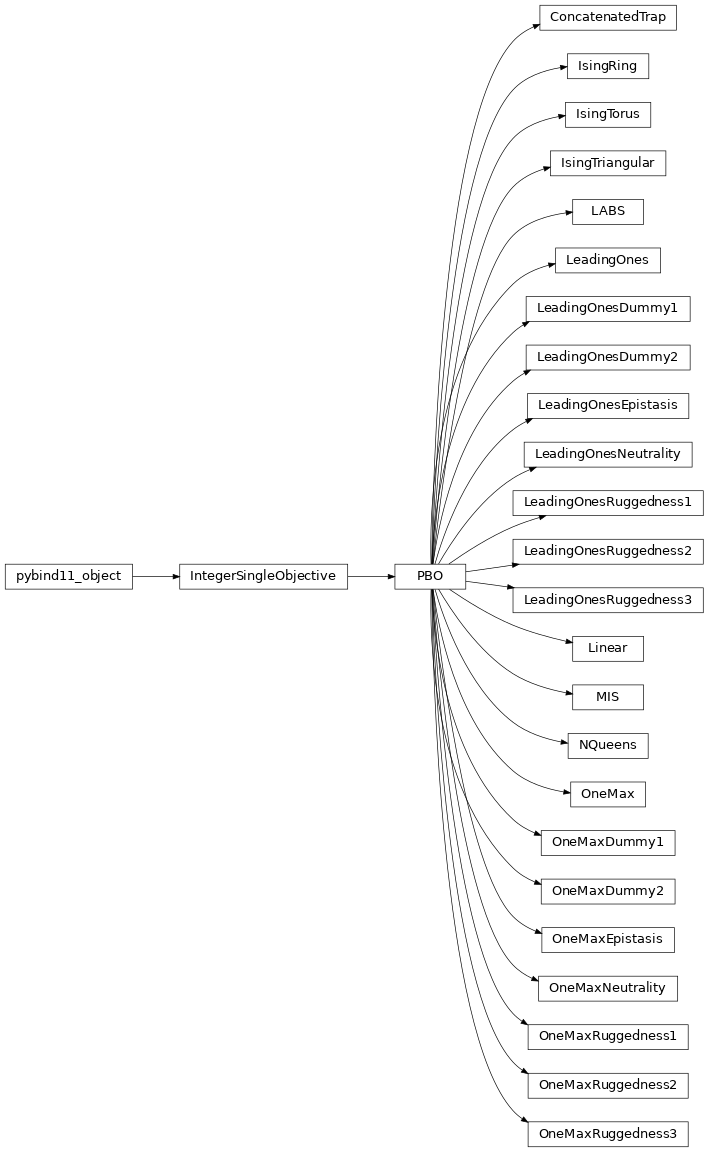# PBO#

Pseudo-Boolean Optimization (PBO) problem set, which contains 25 test functions taking their domain on {0, 1}^n, where n is the length of bitstrings.

In PBO, we cover some theory-motivated function, e.g., OneMax and LeadingOnes as well as others with more practical relevance, e.g., the NK Landscape [DoerrYHWSB20]. We also utilized the so-called W-model for generating/enriching the problem set [WeiseW18].

## Problems#

### Classes#

 `ConcatenatedTrap`(self, instance, n_variables) Concatenated Trap (CT) is defined by partitioning a bit-string into segments of length k and concatenating m = n/k trap functions that takes each segment as input. `IsingRing`(self, instance, n_variables) The Ising Spin Glass model arose in solid-state physics and statistical mechanics, aiming to describe simple interactions within many-particle systems. `IsingTorus`(self, instance, n_variables) The Ising Spin Glass model arose in solid-state physics and statistical mechanics, aiming to describe simple interactions within many-particle systems. `IsingTriangular`(self, instance, n_variables) The Ising Spin Glass model arose in solid-state physics and statistical mechanics, aiming to describe simple interactions within many-particle systems. `LABS`(self, instance, n_variables) Low Autocorrelation Binary Sequences (LABS): x ↦ n^2 / 2∑_{k=1}^{n-1}(∑_{i=1}^{n−k}s_is_{i+k})^2, where s_i = 2x_i − 1 `LeadingOnes`(self, instance, n_variables) LeadingOnes: {0,1}^n → [0..n], x ↦ max{i∈[0..n] ∣ ∀j≤i: x_j=1} `LeadingOnesDummy1`(self, instance, n_variables) A variant of LeadingOnes applying the Dummy transformation of W-model. `LeadingOnesDummy2`(self, instance, n_variables) A variant of LeadingOnes applying the Dummy transformation of W-model. `LeadingOnesEpistasis`(self, instance, n_variables) A variant of LeadingOnes applying the Epistasis transformation of W-model. `LeadingOnesNeutrality`(self, instance, ...) A variant of LeadingOnes applying the Neutrality transformation of W-model. `LeadingOnesRuggedness1`(self, instance, ...) A variant of LeadingOnes applying the first Ruggnedness transformation in https://doi.org/10.1016/j.asoc.2019.106027. `LeadingOnesRuggedness2`(self, instance, ...) A variant of LeadingOnes applying the second Ruggnedness transformation in https://doi.org/10.1016/j.asoc.2019.106027. `LeadingOnesRuggedness3`(self, instance, ...) A variant of LeadingOnes applying the third Ruggnedness transformation in https://doi.org/10.1016/j.asoc.2019.106027. `Linear`(self, instance, n_variables) A Linear Function with Harmonic Weights: {0,1}^n → ℝ, x ↦ ∑_i i * x_i `MIS`(self, instance, n_variables) The maximum independent vertex set (MIVS) formulated as x ↦ ∑_i x_i - n ∑_{i,j} x_i x_j e_{i,j}, where e_{i,j} = 1 if {i,j} in E, otherwise e_{i,j} = 0. `NQueens`(self, instance, n_variables) The N-queens problem (NQP) is defined as the task to place N queens on an N*N chessboard in such a way that they cannot attack each other. `OneMax`(self, instance, n_variables) OneMax: {0,1}^n → [0..n], x ↦ ∑_{i=1}^n x_i. `OneMaxDummy1`(self, instance, n_variables) A variant of OneMax applying the Dummy transformation of W-model. `OneMaxDummy2`(self, instance, n_variables) A variant of OneMax applying the Dummy transformation of W-model. `OneMaxEpistasis`(self, instance, n_variables) A variant of OneMax applying the Epistasis transformation of W-model. `OneMaxNeutrality`(self, instance, n_variables) A variant of OneMax applying the Neutrality transformation of W-model. `OneMaxRuggedness1`(self, instance, n_variables) A variant of OneMax applying the first Ruggnedness transformation in https://doi.org/10.1016/j.asoc.2019.106027. `OneMaxRuggedness2`(self, instance, n_variables) A variant of OneMax applying the second Ruggnedness transformation in https://doi.org/10.1016/j.asoc.2019.106027. `OneMaxRuggedness3`(self, instance, n_variables) A variant of OneMax applying the third Ruggnedness transformation in https://doi.org/10.1016/j.asoc.2019.106027. `PBO` Pseudo-Boolean Optimization (PBO) problem set.

### Class Inheritance Diagram### Reference#

[DoerrYHWSB20] Carola Doerr, Furong Ye, Naama Horesh, Hao Wang, Ofer M. Shir, and Thomas Bäck. “Benchmarking discrete optimization heuristics with IOHprofiler.” Applied Soft Computing 88 (2020): 106027.

[WeiseW18] Thomas Weise and Zijun Wu. “Difficult features of combinatorial optimization problems and the tunable w-model benchmark problem for simulating them.” In Proceedings of the Genetic and Evolutionary Computation Conference Companion, pp. 1769-1776. 2018.